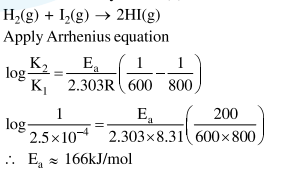# Solve this following

Question:

For the reaction of $\mathrm{H}_{2}$ with $\mathrm{I}_{2}$, the rate constant is $2.5 \times 10^{-4} \mathrm{dm}^{3} \mathrm{~mol}^{-1} \mathrm{~s}^{-1}$ at $327^{\circ} \mathrm{C}$ and $1.0 \mathrm{dm}^{3} \mathrm{~mol}^{-1} \mathrm{~s}^{-1}$ at $527^{\circ} \mathrm{C}$. The activation energy for the reaction, in $\mathrm{kJ} \mathrm{mol}^{-1}$ is: $\left(\mathrm{R}=8.314 \mathrm{~J} \mathrm{~K}^{-1} \mathrm{~mol}^{-1}\right)$

1. 72

2. 166

3. 150

4. 59

Correct Option: , 2

Solution: